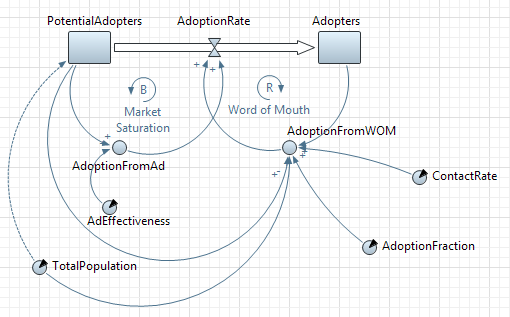AnyLogic

We need to add two dynamic variables representing adoptions resulting from word of mouth and from advertising.

1. Drag the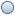Dynamic Variable element from the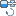System Dynamics palette onto the graphical diagram of agent.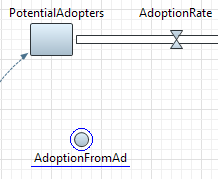Again, as with mentioning some variable in stock’s initial value, when you mention some variable in equation of dynamic variable, flow, or some other system dynamics element, you need to connect those variables with a link prior to specifying the formula.

1. Add a link from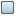PotentialAdopters to AdoptionFromAd.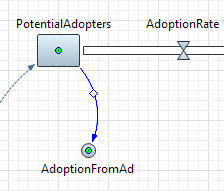2. You can adjust the link appearance (change its arc) by dragging the marker in the middle of the link. Adjust the appearance to make the link look like in the following figure: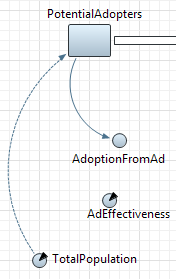You may have noticed that this link looks different from the one drawn from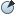TotalPopulation toPotentialAdopters. Links to variables mentioned in stock initial values are drawn with dotted lines, while other links — with solid lines.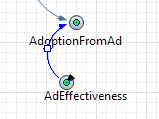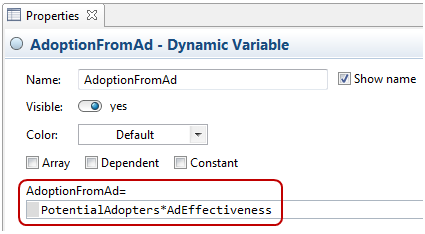1. Add one moreDynamic Variable. Name it AdoptionFromWOM and specify the following formula:
You may find it boring to add numerous links for all the variables mentioned in this formula. For this case AnyLogic provides a quick fix mechanism that automatically creates missing links to the variables mentioned in an equation.
2. After specifying the given formula, click inside the formula field. You will see error indicators.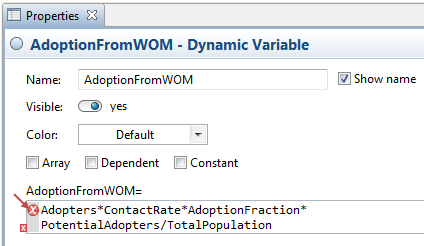3. Click the error indicator (right where the arrow points in the figure above). You will see the context menu with items Create missing link for: ContactRate, etc. Choose this item and the corresponding link from theContactRate parameter to AdoptionFromWOM will be created. Do the same things for all other missing links one by one.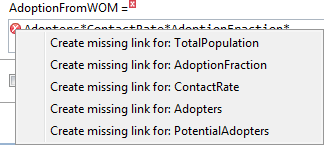Finally you will get the following stock and flow diagram: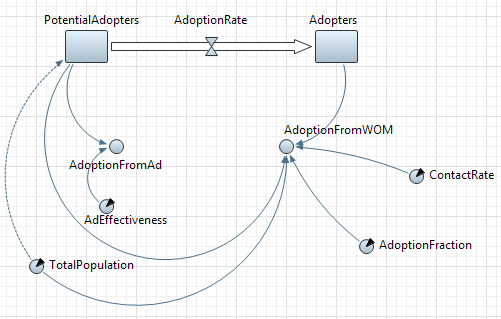Now we can formulate the adoption flow using just defined dynamic variables. The two sources of adoption are assumed to be independent. Thus, the total adoption rate is the sum of adoptions resulting from word of mouth driven by the population of adopters and adoptions resulting from advertising.

Define the formula for the adoption rate

2. Now select the flow on the diagram and go to the flow properties. Specify the formula expression in the AdoptionRate= field: AdoptionFromAd+AdoptionFromWOM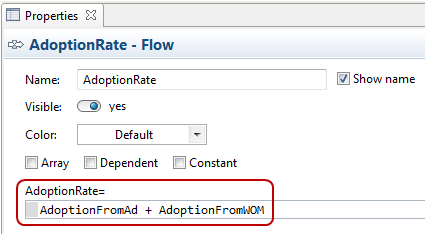Now we have completely defined our model. The stock and flow diagram of the model should look like as on the figure below.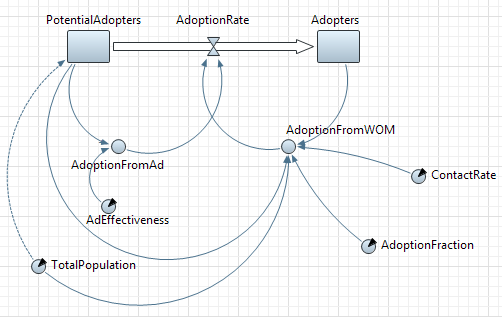The relationships between variables, represented by links, can be labeled as positive or negative.

• Positive causal link means that the two variables change in the same direction, i.e. if the variable in which the link starts decreases, the other variable also decreases. Similarly, if the variable in which the link starts increases, the other variable increases.
• Negative causal link means that the two variables change in opposite directions, i.e. if the variable in which the link starts increases, then the other variable decreases, and vice versa.

Actually all of them are positive except for the one going fromTotalPopulation to AdoptionFromWOM.

1. To add a polarity sign, select the link in the graphical editor and choose + or - option from the Polarity group of options in the link’s Properties.
2. You can change here the color of the link either (in the Color control).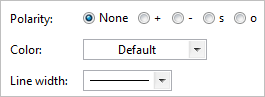Our model has one balancing and one reinforcing feedback loop.

• A balancing feedback loop affects the adoption rate due to advertising. The adoption rate reduces the pool of the potential adopters, which in turn decreases the adoption rate.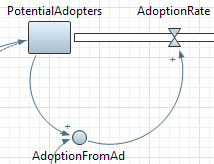• A reinforcing loop affects the adoption rate due to word of mouth. The adoption rate increases the adopter population, resulting in an increase of word of mouth, and thus the increase of the adoption rate.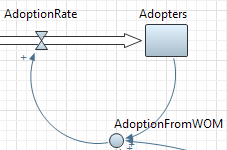Add an identifier for Market Saturation loop

1. Drag the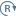Loop element from theSystem Dynamics palette onto the graphical diagram, as shown in the figure below: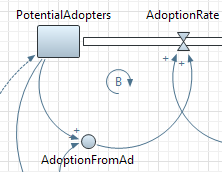2. Go to the Properties view to set up properties of the loop.
3. Set the Direction of this loop — this loop is Counterclockwise.
4. Type the brief text explaining the meaning of this loop in the Text property: Market Saturation. This text will be shown on the presentation.
5. Choose the symbol that will be shown for this element from the Type group of buttons. Choose B here (stands for Balancing) since this loop is balancing.

To determine if a causal loop is reinforcing or balancing, one can start with an assumption, e.g. "VariableN increases" and follow the loop around. The loop is:

• reinforcing if, after going around the loop, one ends up with the same result as the initial assumption.
• balancing if the result contradicts the initial assumption.

Add an identifier for Word of Mouth loop

1. Add one more loop identifier as shown in the figure below: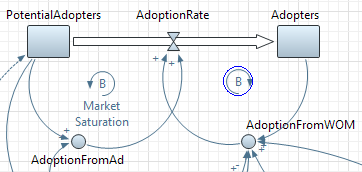2. This loop stands for word of mouth effect. It is reinforcing, so choose R as a symbol denoting its type.
3. Specify Word of Mouth as its Text.
4. Set the Direction of this loop — this loop is Clockwise.

Finally the diagram should look like in the figure below: